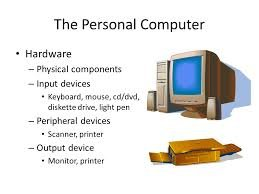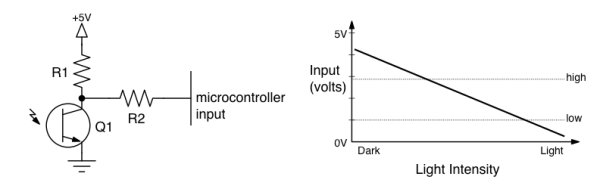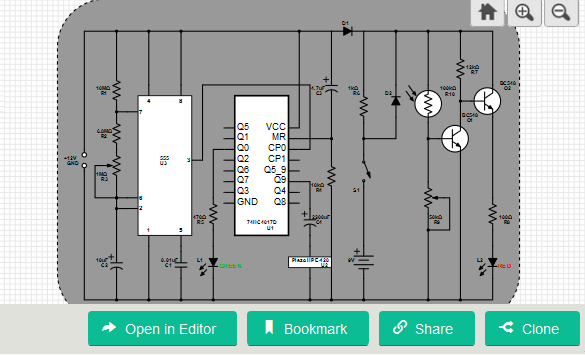# Input programming activity

The previous activities showed you how to make the microcontroller activate outputs. This activity demonstrates how to receive inputs from externally connected devices. The input programs also introduce the concept of abstraction—the ability of a program to refer to I/O circuits using assigned variable names rather than their specific hardware addresses.

## What you should know before starting

### CHRP related information

If you examine the CHRP 2 schematic, you can see that the microcontroller’s Port B pins are connected to both the octal buffer that drives the LEDs, as well as to eight pushbutton switches. You might wonder how the microcontroller can use one I/O port for both output and input circuits at the same time. It’s relatively easy, as it turns out, through a combination of CHRP hardware design and the use of the previously described TRIS registers.First, let’s examine the output part of the hardware. On the schematic, notice that the LEDs are not connected directly to PORTB. Instead, the LEDs are driven through a buffer IC. The buffer presents a high-impedance load to the microcontroller I/O pins. In other words, the buffer just senses the state of the PORTB wires, and then activates the appropriate LEDs—in effect isolating the LEDs from the other PORTB circuitry.

Now, let’s look at the input circuits. The PORTB pushbuttons are connected directly to the PORTB I/O pins, albeit through a series resistor. When the port pin is an input and the pushbutton is pressed, very little current flows between ground and the I/O pin so the series resistor has a negligible effect. If the pushbutton is pressed while the port pin is outputting a high signal, the series resistor limits the current flow to ground, preventing a short circuit on the output.

Port A of the microcontroller also includes some input circuits. Both phototransistors (Q1 and Q2) as well the potentiometer inputs can be sensed by the PIC. These circuits are input only in the CHRP 2 board—Port A is not shared with any output devices. The potentiometer and phototransistor Q1 share one input pin—use J9 to select which one of the two connects to bit 0 of Port A.

### Microcontroller related information

Now, let’s re-examine the function of the TRIS registers. The TRISA and TRISB registers control the direction of data transfer on each pin of Port A and Port B, respectively. The easy way to remember the TRIS register settings is to match the numbers 0 and 1 to the letters O and I:

0 is like O, as in Output
1 is like I, as in Input

### How input circuits work

The CHRP circuit board includes a number of input circuits, such as pushbutton switches, phototransistors (light sensors), and a potentiometer (position sensor). All of these inputs operate in a similar, though slightly different way. Examine the switch input schematic diagram, below:

The switch (S1) and pull-up resistor (R1) form a series circuit, also known as a voltage divider. The microcontroller input is connected to the output at the mid-point of the voltage divider, and senses the electrical potential across the switch. Since any microcontroller pins configured as inputs have a high impedance, they will just sense the externally applied voltage but won’t conduct any appreciable current themselves. This essentially means that the effect of the series resistor (R2) between the voltage divider and the microcontroller is negligible and can be safely ignored—R2 is installed to limit the current due to ESD (electro-static discharge), protecting the microcontroller from static charges conducted into the switch circuit by a user’s fingers.

Ignoring R2, the operation of the switch input circuit can be explained by the interaction of the switch and pull-up resistor R1. You can think about how this works either by Ohm’s law analysis, or by thinking about it as a voltage divider. Using Ohm’s Law analysis, we know that when the switch is open, no current flows through the pull-up resistor. Since no current flows, the voltage loss across the resistor is zero, and the microcontroller input pin senses the full power supply voltage connected to the resistor—5V, or a logic 1. You could say the resistor pulled-up the switch circuit voltage to 5V.When the switch is pressed, current flows through both the switch and resistor. Since the switch has effectively zero resistance, there is a zero volt loss across the switch. Ohm’s Law shows that the current flow through the resistor causes all of the applied voltage to be dissipated across the resistor, and so the microcontroller ends up sensing the ground potential, 0V, or a logic 0.

Thinking about the circuit as a voltage divider, we know that the two elements in a series circuit divide the applied voltage in the ratio of their resistances. The voltage at the mid-point of the switch circuit will be proportional to the resistance of the switch, which has either infinite resistance (switch open), or zero resistance (switch closed). When the switch is open, its infinite resistance is infinitely higher than that of the pull-up resistor, causing the entire applied voltage to be measured across the switch, and producing a logic 1 (5V). When the switch is closed, its (ideally) zero resistance is infinitely smaller than that of the pull-up resistor, causing no voltage drop, and producing a logic 0 (0V).

For more detail: Input programming activity